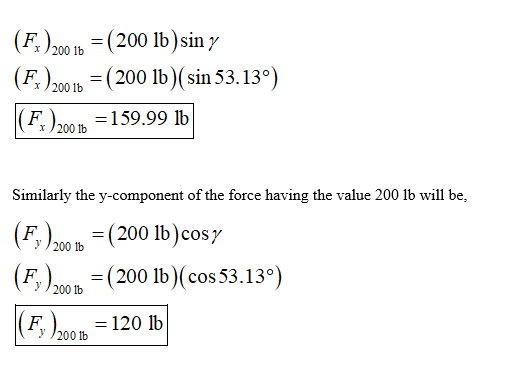# Determine the x and y components of each of the forces shown.

Question-AnswerCategory: Engineering MechanicsDetermine the x and y components of each of the forces shown.

Determine the x and y components of each of the forces shown.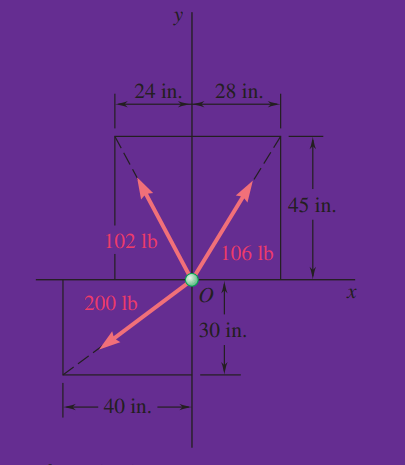Step1
Consider the modified diagram for the provided one.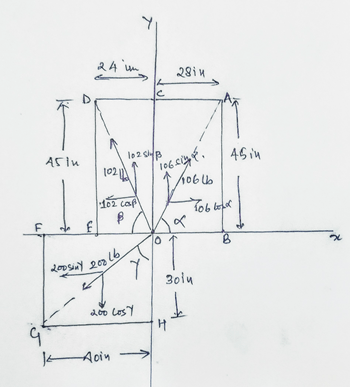Here in the diagram, the angles alpha, beta and gamma are shown, which is the angle between the 106lb force and x-axis, 102 lb fore with the x axis (negative) and 200 lb force with y axis (negative) respectively. The x and y components of all the forces are also shown in the above diagram.

Step 2

The subsequent step is to determine the angles,
For the angle alpha, consider the right angled triangle AOB and apply the trigonometric ratio for the tangent angle,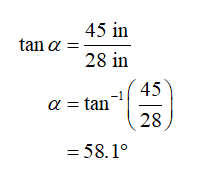Step 3
The x-component of the force having the value 106 lb will be,Step 4

Further, for the angle beta, consider the right angled triangle DOE and apply the trigonometric ratio for the tangent angle,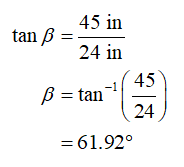Step 5

The x-component of the force having the value 102 lb will be,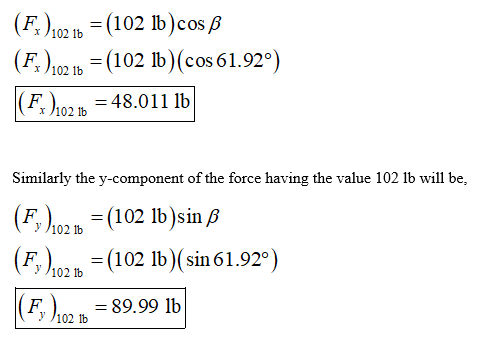Step 6

Finally, for the angle gamma, consider the right angled triangle GOH and apply the trigonometric ratio for the tangent angle,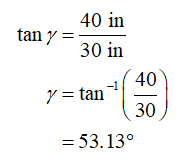Step 7

The x-component of the force having the value 200 lb will be,